Latest Banking jobs   »

# Quantitative Aptitude Quiz For SBI PO/Clerk Mains 2023- 7th January

Q1. A and B are two alloys which were made by mixing iron and copper in the ratio of 3 : 5 and 5 : 9 respectively. If 60 grams of alloy A and X grams of alloy B are melted and mixed to form another alloy C, what is the value of X if the ratio of iron and copper in the new alloy is 35 : 61 ?
(a) 70 gms
(b) 56 gms
(c) 98 gms
(d) 84 gms
(e) 112 gms

Q2. In a class there are two sections i.e. A and B. 25% of the students in section A and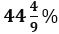of the students in section B are girls. If two students are chosen at random one each from section A and section B as class representative then find the probability that there will be exactly one boy out of chosen two students?
(a) 17/36
(b) 13/36
(c) 1/2
(d) 7/18
(e) 4/9

Q3. Ravi and Sneha got married 10 years ago and at that time ratio of their ages was 5:4. Ratio of present age of Ravi and Sneha is 7:6. After marriage they had seven children including a triplet and a twin. The ratio of present age of triplet, twin, sixth and the seventh child is 4:3:2:1. Find the largest possible value of the present total age of the family.
(a) 121
(b) 107
(c) 101
(d) 93
(e) None of the above

Q4. Shivam invested Rs 3 lac in a scheme which is providing interest rate of ‘r’% per annum at CI and this scheme doubles the sum invested in 72/r years which is two times of rate of interest provided by the scheme. Find the total amount received by Shivam at the end of 48 years (in lac)?
(a) 45
(b) 50
(c) 64
(d) 32
(e) 48

Q5. Marked price of two articles A and B is in the ratio of 3:4. Shopkeeper sold article A and article B at the discount of x % and (x+8)% respectively. Shopkeeper made a profit of 20% on selling article B and found that its cost price is equal to selling price of article A. Find the marked of article B when article A is sold for Rs 972 at two successive discounts of x/2% and 2x%?
(a) Rs 1800
(b) Rs 2000
(c) None of the above
(d) Rs 1600
(e) Rs 2800

Directions (6-10): Bar graph shows total number of students (Medical and Non-medical) in section A and section B in 4 different years. Table has 3 columns, first shows year, column second mention the ratio of student having medical side to that of non-medical side. Column III shows percentage of Medical students in section A, out of total medical students.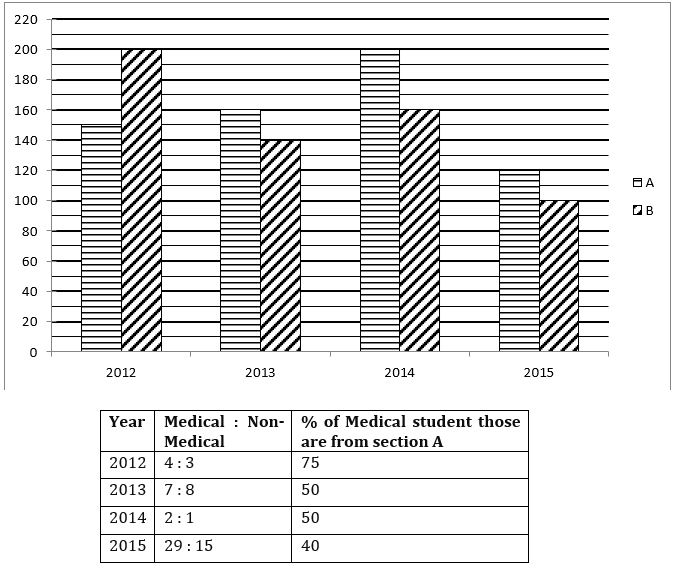Q6. Calculate the percentage of medical student from section B out of total medical students in year 2012.
(a) 0%
(b) 25%
(c) 40%
(d) 50%
(e) None of these

Q7. What is the ratio of medical student of section B to non-medical student of section A of year 2014.
(a) 1 : 1
(b) 2 : 3
(c) 5 : 3
(d) 3 : 2
(e) 2 : 5

Q8. What is the ratio of total number of medical students to total number of non medical students of all these years together?
(a) 145 : 101
(b) 29 : 21
(c) 7 : 5
(d) 4 : 3
(e) None of these

Q9. In year 2013, 10% of those who have medical, qualified PMT & 25% of those who have non-medical qualified JEE, then calculate total percentage of students who either qualified JEE or PMT in year 2013.
(a) 54%
(b) 18%
(c) 20%
(d) 16%
(e) 44%

Q10. What is the % of non-medical students of section B with respect to total students of section B in year 2015.
(a) 21%
(b) 16%
(c) 13%
(d) 17%
(e) 19%

Directions (11-15): What approximate value should come in place of question mark (?)in the following questions?

Q11. 44.04 ÷ 3.97 × 7.49 ÷ 2.54 + 3 = √?
(a) 6
(b) 36
(c) 24
(d) 1296
(e) 216

Q12.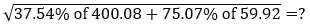(a) 7
(b) 14
(c) 49
(d) 98
(e) 196

Q13.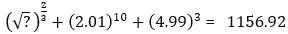(a) 256
(b) 128
(c) 512
(d) 1024
(e) 1296

Q14.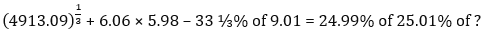(a) 1040
(b) 1600
(c) 400
(d) 800
(e) 1200

Q15. (9.01)³ + (7.94)³ + 5.12 × 17.95 = x³
(a) 11
(b) 15
(c) 13
(d) 10
(e) 14

Solutions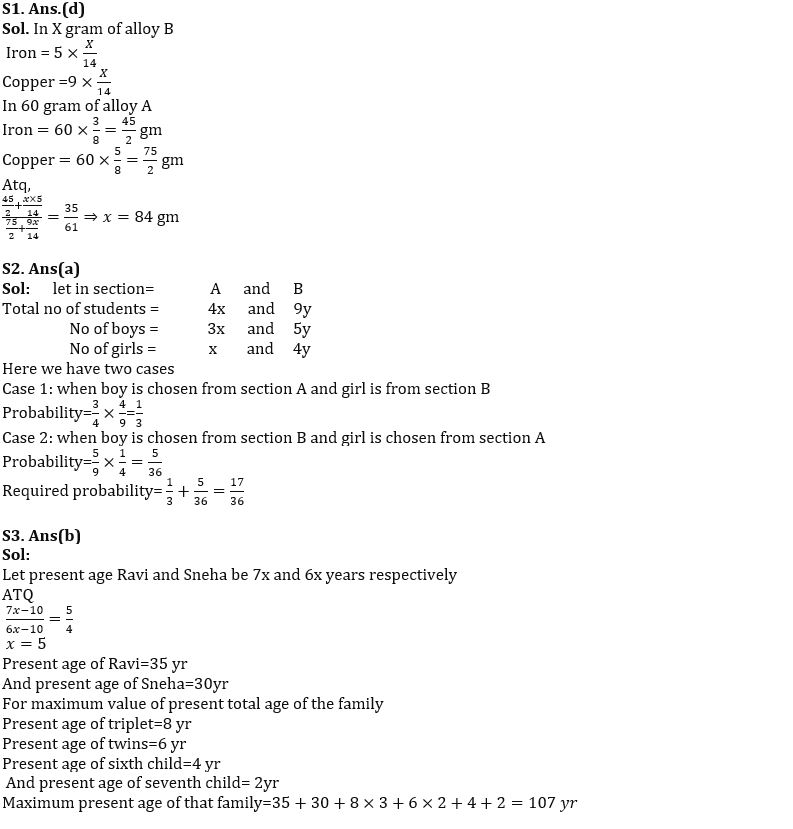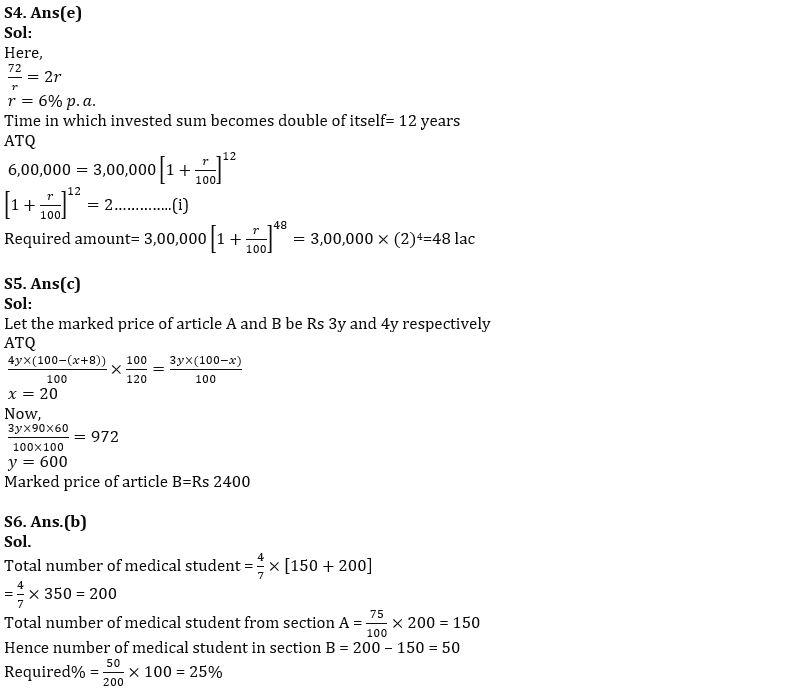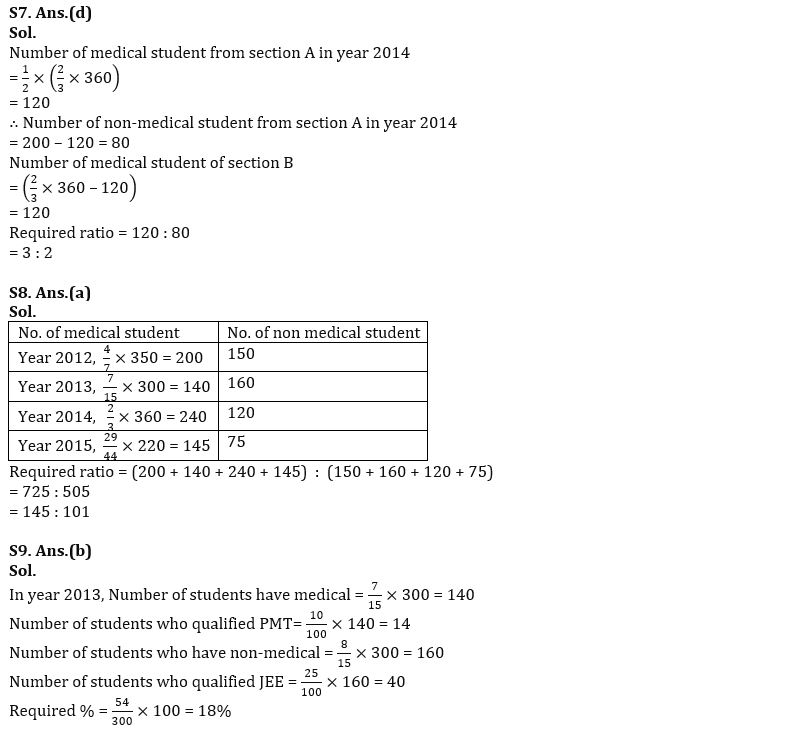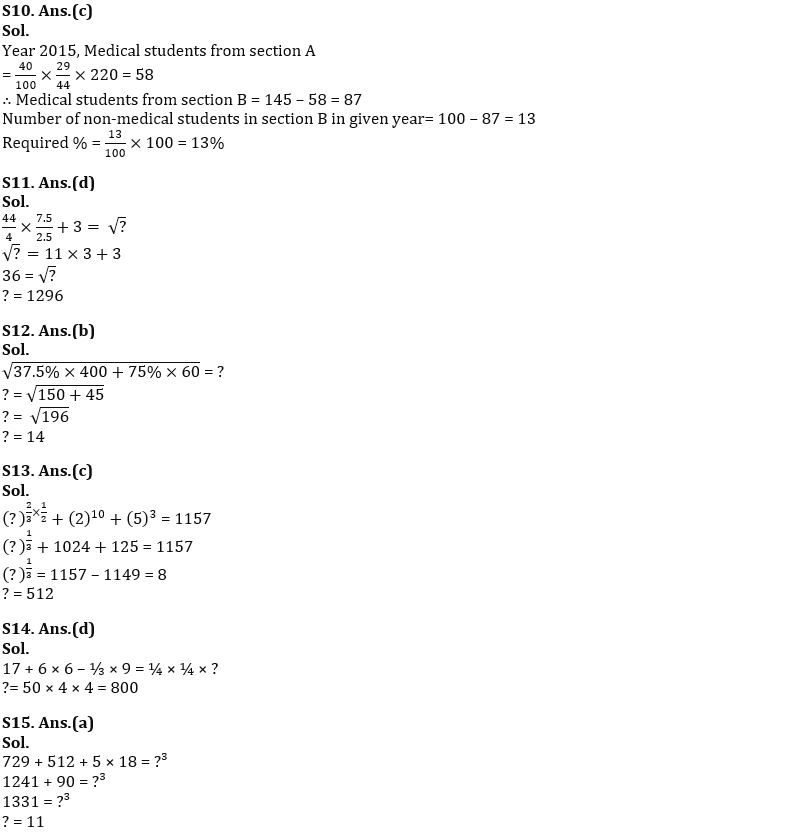## FAQs

### Q1. A and B are two alloys which were made by mixing iron and copper in the ratio of 3 : 5 and 5 : 9 respectively. If 60 grams of alloy A and X grams of alloy B are melted and mixed to form another alloy C, what is the value of X if the ratio of iron and copper in the new alloy is 35 : 61 ? (a) 70 gms (b) 56 gms (c) 98 gms (d) 84 gms (e) 112 gms

S1. Ans.(d)

#### Congratulations!Union Budget 2023-24: Free PDF